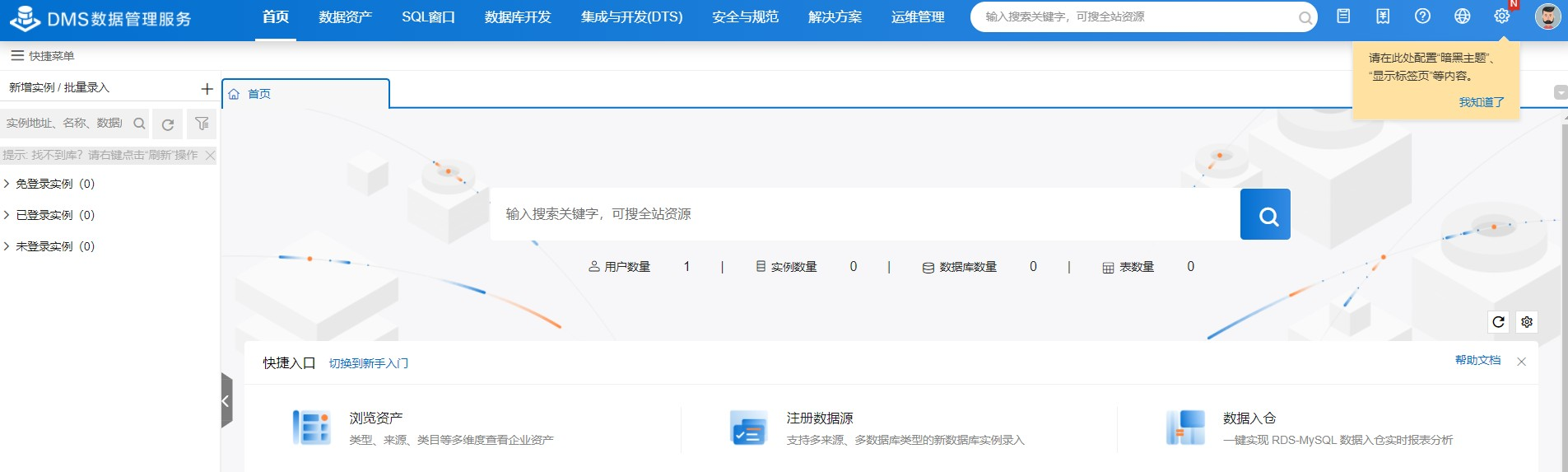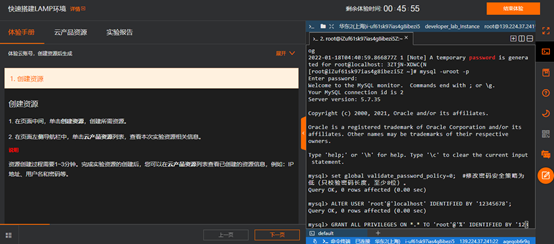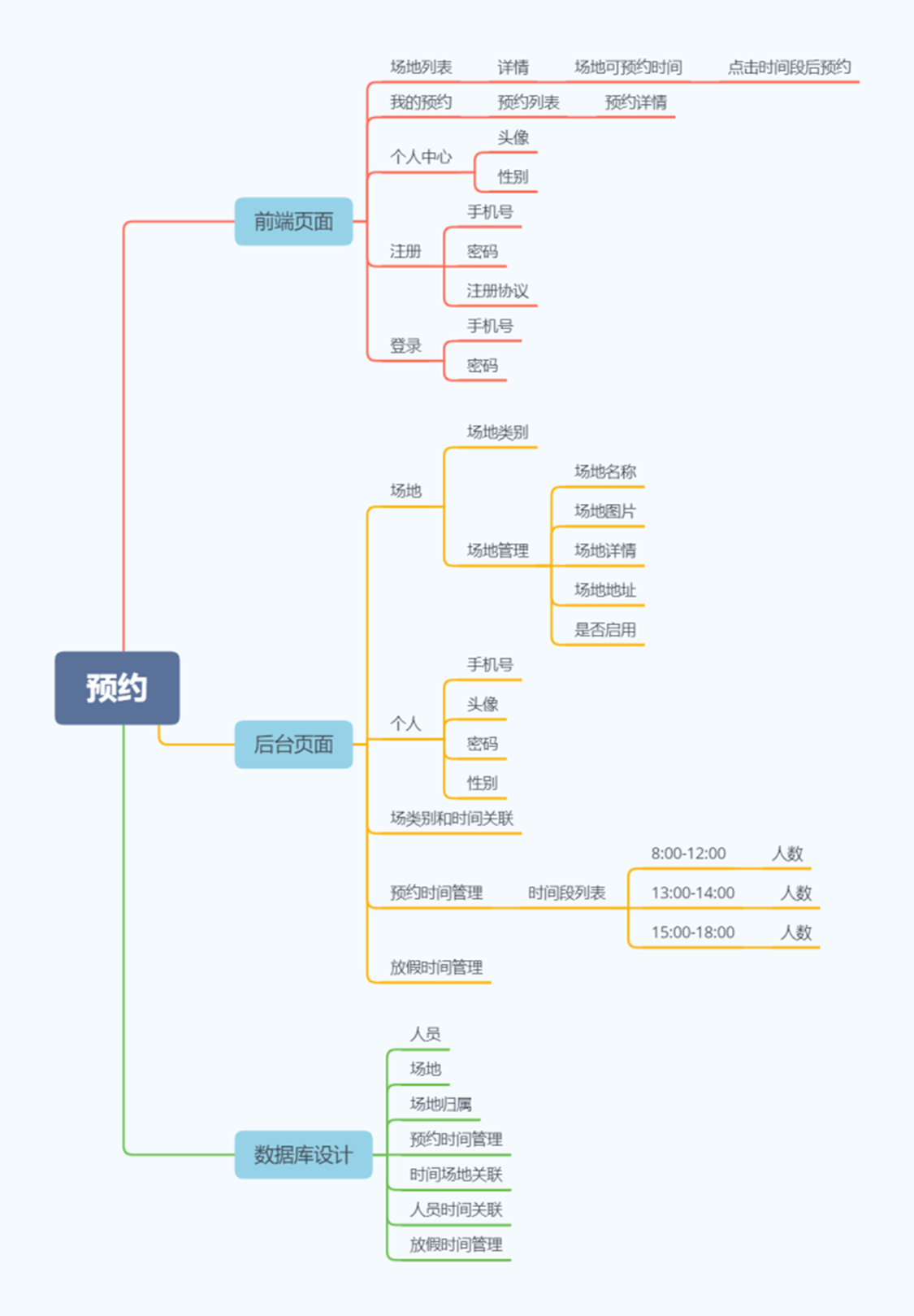php数组函数序列之array_unshift() 在数组开头插入一个或多个元素

+关注继续查看

array_unshift() 函数在数组开头插入一个或多个元素。被加上的元素作为一个整体添加，这些元素在数组中的顺序和在参数中的顺序一样

array_unshift()定义和用法
array_unshift() 函数在数组开头插入一个或多个元素。

array_unshift(array,value1,value2,value3...)参数 描述
array 必需。规定输入的数组。
value1 必需。规定插入的值。
value2 可选。规定插入的值。
value3 可选。规定插入的值。

<?php
$a=array("a"=>"Cat","b"=>"Dog"); array_unshift($a,"Horse");
print_r($a); ?> 输出： Array (  => Horse [a] => Cat [b] => Dog )例子 2 返回键值： 复制代码代码如下: <?php$a=array("a"=>"Cat","b"=>"Dog");
print_r(array_unshift($a,"Horse")); ?> 输出： 3例子 3 数组带有数值键： 复制代码代码如下: <?php$a=array(0=>"Cat",1=>"Dog");
array_unshift($a,"Horse"); print_r($a);
?>

Array (  => Horse  => Cat  => Dog )11 0【在家实践】 ECS 初体验

11 017 016 0DevOps中开发的作用和主动性
DevOps中开发的作用和主动性
3 06 09 06 07 07 0
suboysugar

710

0

《2021云上架构与运维峰会演讲合集》

《零基础CSS入门教程》

《零基础HTML入门教程》